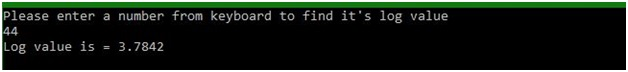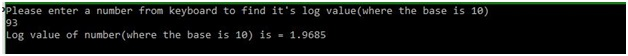# log() and log10() functions of math.h in C

In this article, we are going to learn about the pre-defined functions (log() and log10()) of math.h header file to calculate the log value of any decimal as well as integer value in C programming language.
Submitted by Manu Jemini, on March 17, 2018

If you are making a complex mathematical program than invariably you will need log() and log to the base 10’s value. To make use of these useful functions in your program, you should have math.h file included in your program. That will make these two functions available for us to use.

Now, to use log() function all you will do is pass a number of which you want the value of log. This function will return you the log value of that number.

Similarly, just like log() function, log10() will need a number to be passed as a parameter of which you want the log value. That function will also return a number which can be stored for further usage.

You should know the difference between the log and log10 in mathematics.

## math.h - log() function Example in C

﻿

```#include <stdio.h>
// to use 'log()' function
#include <math.h>

int main()
{
// set the type of variable
float a, num;

// message for user
printf("Please enter a number from keyboard to find it's log value \n");
scanf("%f", &num);

// storing the log value
a = log(num);

// printing the calculated value
printf("Log value is = %.4f\n", a);

return 0;
}
```

Output## math.h - log10() function Example in C

﻿

```#include <stdio.h>
// to use 'log10()' function
#include <math.h>

int main()
{
// set the type of variable
float a, num;

// message for user
printf("Please enter a number from keyboard to find it's log value(where the base is 10) \n");
scanf("%f", &num);

// storing the log value(where the base is 10)
a = log10(num);

// printing the calculated value
printf("Log value of number(where the base is 10) is = %.4f\n", a);

return 0;
}
```

Output